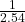## A typical atom has a diameter of about 1.32 x 10-10 m. A) What is this in inches? B) How many atoms are there along a 21.0cm lin

Question

A typical atom has a diameter of about 1.32 x 10-10 m.
A) What is this in inches?
B) How many atoms are there along a 21.0cm line?

in progress 0
2 months 2021-07-30T06:22:47+00:00 1 Answers 4 views 0

A) The value of the diameter in inches is 5.19685 × 10⁻⁹ inches

B)  There are 1,590,909,091 atoms on a 21.0cm line

Explanation:

A) To determine what its diameter is in inches

We will convert 1.32 × 10⁻¹⁰ m to inches

First convert this to centimeters (cm)

1.32 × 10⁻¹⁰m = 1.32 × 10⁻¹⁰ × 10² cm

=  1.32 × 10⁻⁸cm

Now, since 1 inch = 2.54 cm

∴ 1 cm =inches

If  1 cm =inches

Then 1.32 × 10⁻⁸ cm will be

1.32 × 10⁻⁸ ×inches

= 5.19685 × 10⁻⁹ inches

This is the value of the diameter in inches

B) For the number of atoms on a 21.0cm line

The diameter of 1 atom is 1.32 × 10⁻¹⁰m

1.32 × 10⁻¹⁰m = 1.32 × 10⁻⁸cm

Hence, on a 21.0 cm line, we will have

(21.0 / 1.32 × 10⁻⁸) atoms

= 1590909091 atoms

Hence, there are 1,590,909,091 atoms on a 21.0cm line